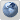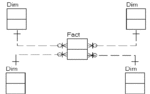Kimball Forum
Would you like to react to this message? Create an account in a few clicks or log in to continue.

# Indicator Dimension or Fact Table

3 posters##Indicator Dimension or Fact Table

Sorry, this is a long explanation but I wanted to document the entire case. Thanks in advance for your advice.

Background:

Currently we are modeling a data mart for traffic accident data. The source system is a repository rather than a transactional system (consolidating data from several sources) and the data is partially denormalized already, with the exception of 10 or so code intensive tables that describe things like, conditions (weather, road, etc), series of events, damages, drug tests and results, etc. I say these tables are code intensive because, in some instances, the tables have over 25 available codes where users can select any combination and number of those codes. Side note - Among the tables codes there is very little overlap (few commonly used codes among the tables; the tables cannot be consolidated).

In current reports (against production repository) these codes are often used as query conditions but also used as aggregates (converting original code values to 1 or 0 using DECODE or CASE) and summed or averaged. We have already decided these tables must be flattened to meet the proper granularity, thus each code will have its own column (Yes 100s of new columns). In standard method, each field will then be populated with an indicator to signify if a value exists (Boolean). Side note - each parent record has many rows in the indicator intensive tables. Instances where no rows exist are rare. Additionally, many records share common indicator patterns.

We do not need to consider any complexities of a Bi layer. Reports are created using Crystal Reports where Crystal points to data in the Warehouse thus, queries of DW data are essentially written manually. Side Note: In queries, the tables/data in question are used in less than ¼ of all standard reports.

The Debate:

Should our new columns utilize 1/0 or Y/N (Boolean number or text) logic to indicate a value exists in the column on the row? Its a given that, if Y/N is the choice then these columns should reside in an indicator dimension but, if 1/0 is chosen, should the columns go to the fact table? After all, if 1/0 is chosen users will be using these values in sum and average functions but also using them as indicators. Side note - None of the fact tables would exceed 60 columns if the indicators were put into the fact tables.

On the users end, the 1/0 logic, if properly used in a query, performs much better than Y/N logic (we will have millions of rows in the associated fact tables). This on its own could justify 1/0, if the incremental gain in performance is significant. An example of the SQL logic is (assuming these tables have already been flattened):

Y/N Logic with an indicator dimension:

SELECT SUM(DECODE (b.code10,Y,1,0)), AVG(a.speed)
FROM fact_table1 a, dimension_table1 b
WHERE (a.sid = b.sid
AND b.code1 = Y
AND b.code2 = Y
AND b.code3 = Y
AND b.code 4 = Y
AND b.code5 = Y
AND b.code6 = N)
OR (b.code1 = N
AND b.code2 = Y
AND b.code3 = Y
AND b.code 4 = Y
AND b.code5 = Y
AND b.code6 = Y)

1/0 Logic where indicators reside in the fact:

SELECT SUM(code10), AVG(speed)
FROM table
WHERE ((code1 + code2 + code3 + code4 + code5) = 5
AND code6 = 0)
OR ((code2 + code3 + code4 + code5 + code6) = 5
AND code61= 0)

If the indicator is Y/N the query becomes long and costly, especially where many complex conditions apply. Additionally, users will frequently be wanting to find counts and averages of event occurrences. If Y/N is the choice SELECT logic will often require complexities. If the indicator is 1/0 the query is extremely simple but, the columns value is used as an aggregate and non-aggregate. Additionally, by having the indicators derived as 1 and 0 in the fact the SELECT logic becomes much more simple - not requiring a CASE or DECODE.

Closing:

In many instance I have used 1/0 logic and placed those columns in the fact table (sometimes this has created a very wide fact table  around 100+ columns). We have then used the columns for both additive and non-additive purposes. These were MY data warehouses where the reporting team was the only users and understood the logic. (The reports team eventually preferred this method over schemas using indicator dimensions and they indicated better performance also). This is NOT my data warehouse and it needs to conform to best practices.

So, considering the factors of this case, what is best practice? Should we stick with the commonly used indicator dimension or should the indicators be derived into 1/0 and placed in the fact table?

Thanks for taking the time to read this,
Andy

kdot

Posts : 3
Join date : 2010-07-20

##Re: Indicator Dimension or Fact Table

Best practice is to use a dimension regardless of the data type of the indicator.

You may also want to consider using a bridge table (multivalued dimension). It doesn't make the queries any simpler but does allow you to add new classifications without modifying the schema.ngalemmo

Posts : 3000
Join date : 2009-05-15
Location : Los Angeles##Re: Indicator Dimension or Fact Table

I set my indicators to (0,1) regardless of whether I perform math operations on them. For your case, it sounds like you could go either way, create a bucket (metric) for each type of accident, or create one metric with the filtering done on the dimension(s). Look at the problem holistically (reuse, other facts, etc) and use that as a guide for the final design.BoxesAndLines

Posts : 1212
Join date : 2009-02-03
Location : USA

##Re: Indicator Dimension or Fact Table

ngalemmo wrote:Best practice is to use a dimension regardless of the data type of the indicator.

You may also want to consider using a bridge table (multivalued dimension). It doesn't make the queries any simpler but does allow you to add new classifications without modifying the schema.

The use of bridge tables is currently being considered though, my thoughts are that this might make the model exceedingly complex since there are 10 or so indicator tables that need modeled (even if consolidated bridge tables are used we will require at least 4  one per grain). My concern is usability, and creating excessive tables [bridge tables] will add complexities for the users. Keeping in mind we dont use a BI layer. Your thoughts?

kdot

Posts : 3
Join date : 2010-07-20

##Re: Indicator Dimension or Fact Table

You can hide the bridge table in views, but I would agree, without a BI layer it would be difficult for a non-technical end-user.ngalemmo

Posts : 3000
Join date : 2009-05-15
Location : Los Angeles##Re: Indicator Dimension or Fact Table